# Dunnett’s Test / Dunnett’s Method: Definition

Share on

## What is Dunnett’s Test?

Dunnett’s Test (also called Dunnett’s Method or Dunnett’s Multiple Comparison) compares means from several experimental groups against a control group mean to see is there is a difference. When an ANOVA test has significant findings, it doesn’t report which pairs of means are different. Dunnett’s can be used after the ANOVA has been run to identify the pairs with significant differences.

One fixed “control” group is compared to all of the other samples, so it should only be used when you have a control group. If you don’t have a control group, use Tukey’s Test.

## Calculation

As Dunnett’s compares two groups, it acts similarly to a t-test. The following formula gives you a value that you can use to compare mean differences.The formula is: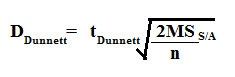Need help? Check out our tutoring page!

Step 1: Look up the tDunnett critical value in the Dunnett-critical value table. You’ll need:

For example, let’s say you had an alpha level of 5%, a sample size of 5 and 20 df. The Dunnett critical value is 2.65.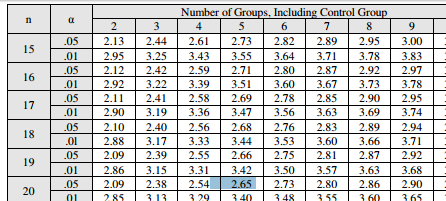Plug the value into the formula: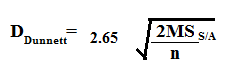Step 2: Find the mean squares (MS) of the within group in the ANOVA source table. Plug that value into the above formula. For example, let’s say your MS within is 15.39: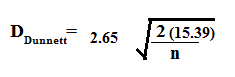Step 3: Find “n”, the number in one group. For this example, that’s 5: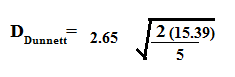Solving the formula, we get:
DDunnett = 2.65 * 2.481 = 6.575.

The answer (6.575) is the critical distance between means. If the distance between a control group mean and an experimental group mean is greater than 6.575, then that distance is significant. For example, let’s say you had a control group mean of 52 and three experimental group means of 55, 64 and 72. The differences between means (absolute values) are:

• Group 1 55 – 52 = 3
• Group 2 64 – 52 = 12
• Group 3 72 – 52 = 20

Only groups 2 and 3 exceed the Dunnett’s distance of 6.575, so they are the significant pairs.

## References

Upton G. & Cook I. (2006.) A Dictionary of Statistics, 2nd edition, Oxford University Press, Oxford, United Kingdom.
Everitt B. S. & Shrondal A. (2010.) The Cambridge Dictionary of Statistics, 4th edition, Cambridge University Press, Cambridge, United Kingdom
Rumsey, Deborah (2009). Statistics II for Dummies. Wiley.

CITE THIS AS:
Stephanie Glen. "Dunnett’s Test / Dunnett’s Method: Definition" From StatisticsHowTo.com: Elementary Statistics for the rest of us! https://www.statisticshowto.com/dunnetts-test/
---------------------------------------------------------------------------Need help with a homework or test question? With Chegg Study, you can get step-by-step solutions to your questions from an expert in the field. Your first 30 minutes with a Chegg tutor is free!